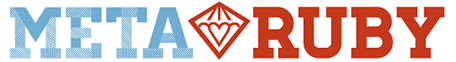# Trying to understand the 2 dotted notation(or 3 dotted) in Ruby

I have to check if my variable idx is in the range between 0 and 8 (both 0 and 8 should be included)
The following comparison works idx<=8 && idx>=0
But I would like to use the dotted syntax instead idx === (0…8) - however it doesn’t work
I was wondering if anybody can see any difference between the two

Below is my function as I am using it in my program

``````def valid_move?(board, idx)
idx<=8 && idx>=0 &&!(position_taken?(board,idx))
end
``````

vs

``````def valid_move?(board, idx)
idx === (0..8) && !(position_taken?(board,idx))
end
``````

Thanks

8 ist a number, 0…8 is a collection of numbers, so you need to check if 8 is a member of 0…8. as far as I remember it’s `(0..8).contains(8)`.

Hi Marin, you should put the Range ahead the idx var:

`(0..8) === idx`

That because of the === operator, changes meaning with the object’s class which is calling it.

For instance `Range.===(Fixnum)` works because in the Range class this operator has been implemented to support a comparison with a Fixnum object.

`Fixnum.===(Range)` doesn’t work because in the Fixnum class, nothing has been implemented to make this operator works with a Range object

I would suggest to use an irb shell, to learn about this and other methods and operators1 Like

So the following code puts true for idx = 1 and 8 and false for idx=9

``````idx=8
if (1..8) === idx
true
else
false
end
# => true``````

That totally worked! Brilliant! Thanks!!!

1 Like